# IIR Halfband Interpolator

Interpolate signal using polyphase IIR halfband filter

•Libraries:
DSP System Toolbox / Filtering / Multirate Filters

## Description

The IIR Halfband Interpolator block performs efficient polyphase interpolation of the input signal by a factor of 2. To design the halfband filter, you can use an elliptic design or a quasi-linear phase design. The block uses these design methods to compute the filter coefficients. To filter the inputs, the block uses a polyphase structure. The allpass filters in the polyphase structure are in a minimum multiplier form.

Elliptic design introduces nonlinear phase and creates the filter using fewer coefficients than the quasi-linear design. Quasi-linear phase design overcomes phase nonlinearity at the cost of additional coefficients.

Alternatively, instead of designing the halfband filter using a design method, you can specify the filter coefficients directly. When you choose this option, the allpass filters in the two branches of the polyphase implementation can be in a minimum multiplier form or a wave digital form.

You can also use the block to implement the synthesis portion of a two-band filter bank to synthesize a signal from lowpass and highpass subbands.

## Examples

expand all

Design and implement an IIR halfband interpolator using the IIR Halfband Interpolator block. Pass a noisy input through the interpolator. Plot the spectrum of the input and the interpolated output in the spectrum analyzer.

Open and inspect the model. The input is a noisy sinusoidal signal with two frequencies, one at 1 kHz and the other at 15 kHz. Add white Gaussian noise with a mean of 0 and a variance of 0.05 to this signal.

The IIR halfband interpolator has a transition width of 4.1 kHz and a stopband attenuation of 80 dB. Visualize the magnitude response of the filter by clicking the View Filter Response button in the block dialog box.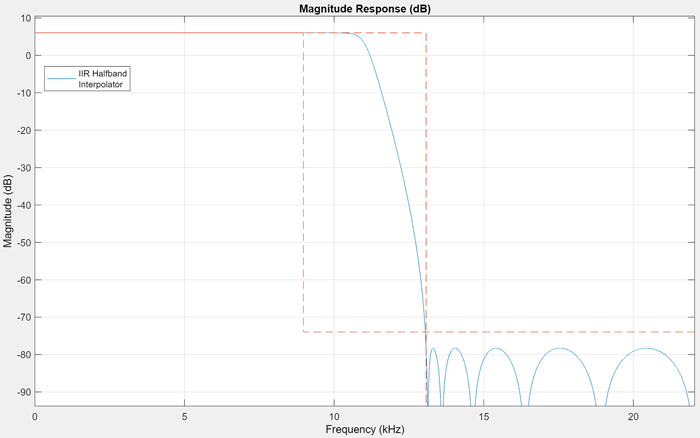Pass the noisy sinusoidal signal through the interpolator. Plot the spectrum of the input and the interpolated output in the spectrum analyzer.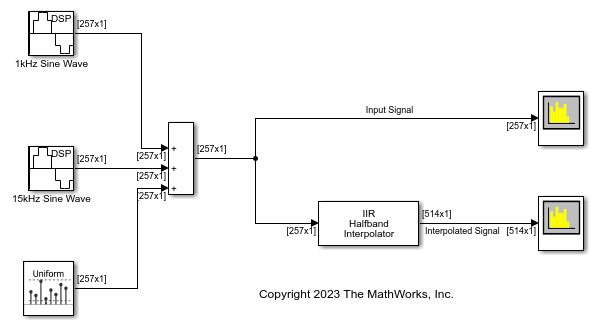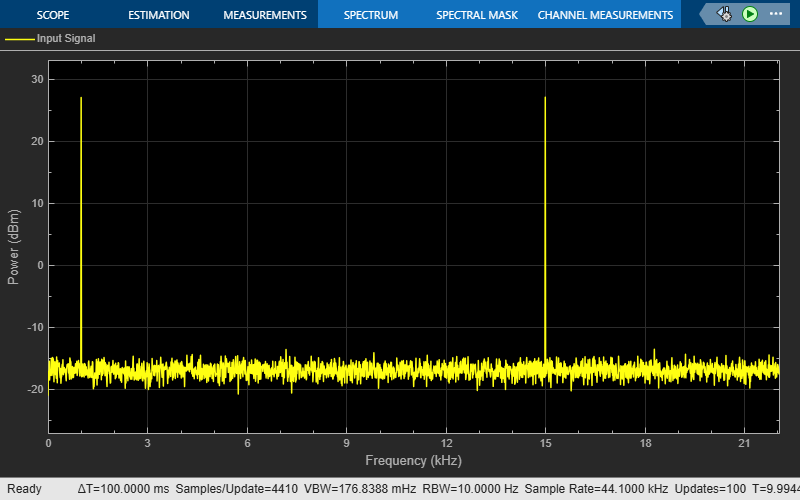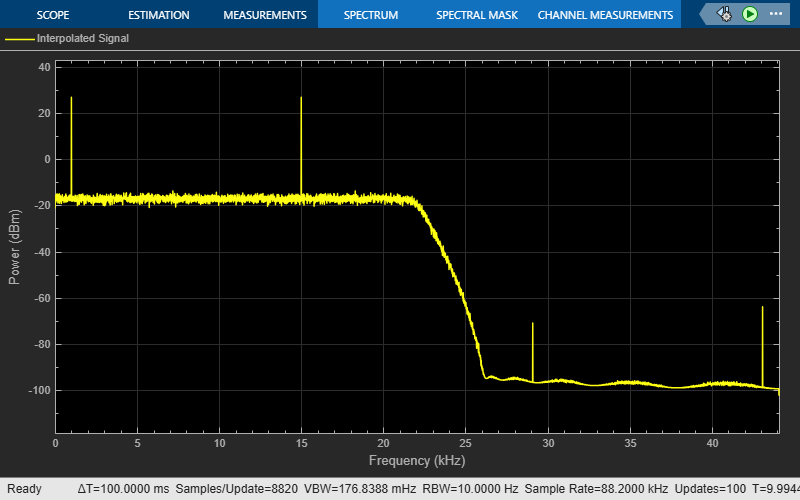Since R2023b

Use the IIR Halfband Decimator and IIR Halfband Interpolator blocks to extract and reconstruct the low-frequency subband from a speech signal.

Open and inspect the model. The input audio data is a single-channel speech signal with the sample rate of 22050 Hz.

Specify the Sample rate mode parameter of the IIR Halfband Decimator and IIR Halfband Interpolator blocks to `Use normalized frequency (0 to 1)`. This option enables you to specify the transition width of the decimation and interpolation filters in normalized frequency units. Set the transition width to 0.093 in normalized frequency units and the stopband attenuation to 80 dB. The design method is `Elliptic` by default.

Read the speech signal from the audio file in frames of 1024 samples. The IIR Halfband Decimator block extracts and outputs the lowpass subband of the speech signal. The IIR Halfband Interpolator block reconstructs the lowpass approximation of the speech signal by interpolating the lowpass subband.

The Audio Device Writer block plays the filtered output.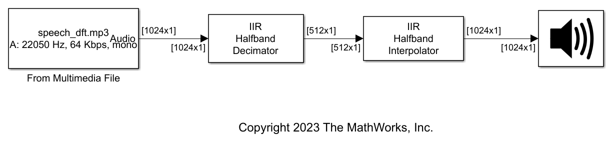Use the IIR Halfband Decimator and IIR Halfband Interpolator blocks to implement a two-channel filter bank. This example uses an audio file input and shows that the power spectrum of the filter bank output does not differ significantly from the input. Play the output of the filter bank using the Audio Device Writer block.

Open and inspect the model. The input audio data is a single-channel speech signal with a sample rate of 22050 Hz.

The IIR Halfband Decimator block acts as an IIR halfband analysis bank as the Output highpass subband parameter is selected in the block dialog box. The IIR Halfband Interpolator block acts as an IIR halfband synthesis bank as the Input highpass subband parameter is selected in the block dialog box.

Set the Sample rate mode parameter in the IIR Halfband Decimator and IIR Halfband Interpolator blocks to `Inherit from input port` so that the blocks inherit the sample rate from the respective input ports. Set the transition width to 4.1 kHz and the stopband attenuation to 80 dB. The design method is set to `Elliptic` by default.

Read the speech signal from the audio file in frames of 1024 samples. The IIR halfband analysis filter bank extracts the lowpass and highpass subbands of the speech signal. The IIR halfband synthesis filter bank synthesizes the speech signal from the lowpass and highpass subbands.

Display the power spectrum of the audio input and the output from the synthesis filter bank in the spectrum analyzer. Play the synthesized speech signal using the Audio Device Writer block.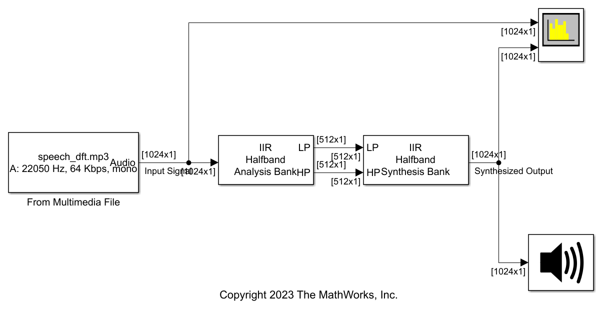## Ports

### Input

expand all

Specify the data input as a vector or a matrix. If the input signal is a matrix, the block treats each column of the matrix as an independent channel.

When you select the Input highpass subband parameter, this block acts as a halfband synthesis filter bank. The input at this port is then the lowpass subband output of a halfband analysis filter bank.

This port is unnamed until you select the Input highpass subband parameter.

Data Types: `single` | `double`
Complex Number Support: Yes

Specify the second input to the synthesis filter bank as a column vector or a matrix. This signal is the highpass subband output of a halfband analysis filter bank. If the input signal is a matrix, the block treats each column of the matrix as an independent channel.

The size, data type, and complexity of both the inputs must be the same.

#### Dependency

To enable this port, select the Input highpass subband parameter.

Data Types: `single` | `double`
Complex Number Support: Yes

Specify the allpass polynomial filter coefficients of the first branch as an N-by-`1` vector or N-by-`2` matrix of N first-order or second-order allpass sections.

#### Dependencies

To enable this parameter, set:

• Filter specification to `Coefficients`

• Internal allpass structure to `Minimum multiplier`

• Clear the Make the first branch a pure delay parameter

• Select the Specify coefficients from input port parameter

Data Types: `single` | `double`

Specify the allpass polynomial filter coefficients of the second branch as an N-by-`1` vector or N-by-`2` matrix of N first-order or second-order allpass sections.

#### Dependencies

To enable this parameter, set:

• Filter specification to `Coefficients`

• Internal allpass structure to `Minimum multiplier`

• Select the Specify coefficients from input port parameter

Data Types: `single` | `double`

### Output

expand all

Output of the interpolator, returned as a column vector or a matrix. The number of rows in the interpolator output is twice the number of rows in the input signal.

Data Types: `single` | `double`

## Parameters

expand all

Select the parameters that the block uses to design the IIR halfband filter. Because the filter design has only two degrees of freedom, you can specify only two of the three parameters:

• ```Transition width and stopband attenuation``` (default) — Design the filter using Transition width (Hz) and Stopband attenuation (dB). This design is the minimum order design.

• `Filter order and transition width` — Design the filter using Filter order and Transition width (Hz).

• ```Filter order and stopband attenuation``` — Design the filter using Filter order and Stopband attenuation (dB).

• `Coefficients`— Specify the filter coefficients directly using the enabled parameters.

Specify the transition width of the IIR halfband filter as a positive scalar in Hz or in normalized frequency units (since R2023b).

If you set the Sample rate mode parameter to:

• `Specify on dialog` or `Inherit from input port` –– The value of the transition width is in Hz and must be less than half the value of the output sample rate (2 × input sample rate).

• `Use normalized frequency (0 to 1)` –– The value of the transition width is in normalized frequency units. The value must be a positive scalar less than `1.0`.

(since R2023b)

#### Dependencies

To enable this parameter, set Filter specification to ```Filter order and transition width``` or ```Transition width and stopband attenuation```.

Specify the filter order as a positive integer. If you set Design method to `Elliptic`, then Filter order must be an odd integer greater than one. If you set Design method to ```Quasi-linear phase```, then Filter order must be a multiple of four.

#### Dependencies

To enable this parameter, set Filter specification to ```Filter order and transition width``` or ```Filter order and stopband attenuation```.

Specify the minimum attenuation needed in the stopband of the IIR halfband filter as a real positive scalar in dB.

#### Dependencies

To enable this parameter, set Filter specification to ```Filter order and stopband attenuation``` or ```Transition width and stopband attenuation```.

Specify the design method for the IIR halfband filter.

• `Elliptic` (default) — The filter has a nonlinear phase and uses few coefficients.

• `Quasi-linear phase` — The first branch of the polyphase filter structure is a pure delay, which results in an approximately linear phase response.

#### Dependencies

To enable this parameter, set Filter specification to any option except `Coefficients`.

Specify the internal allpass filter implementation structure as `Minimum multiplier` or ```Wave Digital Filter```. Each structure uses a different coefficients set, independently stored in the corresponding coefficients property. The default is ```Minimum multiplier```.

#### Dependencies

To enable this parameter, set Filter specification to `Coefficients`.

When you select this check box, the first branch of the polyphase filter structure becomes a pure delay, and the Branch 1 allpass polynomial coefficients and Branch 1 Wave Digital coefficients parameters do not apply.

By default, this check box is not selected.

#### Dependencies

To enable this parameter, set Filter specification to `Coefficients`.

Specify the length of the first branch delay as a finite positive scalar. The default is `1`.

#### Dependencies

To enable this parameter, set:

• Filter specification to `Coefficients`

• Select the Make the first branch a pure delay parameter

When you select this check box, you can input the branch 1 allpass polynomial coefficients and branch 2 allpass polynomial coefficients through the input ports coeffs1 and coeffs2. When you clear this check box, you specify the coefficients in the block dialog box through the Branch 1 allpass polynomial coefficients and Branch 2 allpass polynomial coefficients parameters.

#### Dependencies

To enable this parameter, set:

• Filter specification to `Coefficients`

• Internal allpass structure to `Minimum multiplier`

Specify the allpass polynomial filter coefficients of the first branch as an N-by-`1` vector or N-by-`2` matrix of N first-order or second-order allpass sections.

This parameter is tunable, that is, you can change its value during simulation.

#### Dependencies

To enable this parameter, set:

• Filter specification to `Coefficients`

• Internal allpass structure to `Minimum multiplier`

• Clear the Make the first branch a pure delay parameter

• Clear the Specify coefficients from input port parameter

Specify the allpass polynomial filter coefficients of the second branch as an N-by-`1` vector or N-by-`2` matrix of N first-order or second-order allpass sections.

This parameter is tunable, that is, you can change its value during simulation.

#### Dependencies

To enable this parameter, set:

• Filter specification to `Coefficients`

• Internal allpass structure to `Minimum multiplier`

• Clear the Specify coefficients from input port parameter

Specify the allpass filter coefficients of the first branch in wave digital filter (WDF) form as an N-by-`1` vector or N-by-`2` matrix of N first-order or second-order allpass sections.

The magnitude of each WDF coefficient must not be greater than 1.

#### Dependencies

To enable this parameter, set:

• Filter specification to `Coefficients`

• Internal allpass structure to `Wave Digital Filter`

• Clear the Make the first branch a pure delay parameter

Specify the allpass filter coefficients of the second branch in wave digital filter (WDF) form as an N-by-`1` vector or N-by-`2` matrix of N first-order or second-order allpass sections.

The magnitude of each WDF coefficient must not be greater than 1.

#### Dependencies

To enable this parameter, set:

• Filter specification to `Coefficients`

• Internal allpass structure to `Wave Digital Filter`

When you select this check box, the block treats the last section of the second branch as a first order section. When the coefficients of the second branch are in an N-by-`2` matrix, the block ignores the second element of the last row of the matrix. The last section of the second branch then becomes a first-order section.

When you clear this check box, the block treats the last section of the second branch as a second-order section. When the coefficients of the second branch are in an N-by-`1` matrix, the block ignores this parameter.

By default, this check box is cleared.

#### Dependencies

To enable this parameter, set Filter specification to `Coefficients`.

When you select this check box, the block acts as a synthesis filter bank. The block accepts two inputs to synthesize: lowpass and highpass subbands. When you clear this check box, the block acts as an IIR halfband interpolator and accepts a single vector or matrix as input. By default, this check box is cleared.

Since R2023b

Specify the input sample rate using one of these options:

• `Specify on dialog` –– Specify the input sample rate in the block dialog box using the Input sample rate (Hz) parameter.

• `Inherit from input port` –– The block inherits the sample rate from the input signal.

• `Use normalized frequency (0 to 1)` –– Specify the passband-edge and the stopband-edge frequencies in normalized frequency units (0 to 1).

#### Dependencies

To enable this parameter, set Filter specification to any value other than `Coefficients`.

Specify the sample rate of the input signal as a scalar in Hz.

#### Dependencies

To enable this parameter, set:

• Filter specification to any value other than `Coefficients`.

• Sample rate mode to `Specify on dialog`.

(since R2023b)

Click this button to open the Filter Visualization Tool (FVTool) and display the magnitude and phase response of the IIR Halfband Interpolator. The response is based on the values you specify in the block parameters dialog box. Changes made to these parameters update FVTool.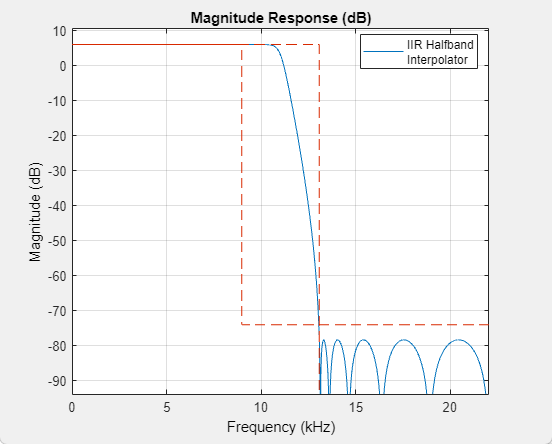To update the magnitude response while FVTool is running, modify the dialog box parameters and click .

Specify the type of simulation to run. You can set this parameter to:

• `Code generation` (default)

Simulate model using generated C code. The first time you run a simulation, Simulink® generates C code for the block. The C code is reused for subsequent simulations, as long as the model does not change. This option requires additional startup time but provides faster simulation speed than ```Interpreted execution```.

• `Interpreted execution`

Simulate model using the MATLAB®  interpreter. This option shortens startup time but has slower simulation speed than ```Code generation```.

## Block Characteristics

 Data Types `double` | `single` Direct Feedthrough `no` Multidimensional Signals `no` Variable-Size Signals `yes` Zero-Crossing Detection `no`

expand all

## Version History

Introduced in R2015b

expand all